Engineering Entrance Sample Papers

GATE Mechanical Engineering sample paperQuestions carrying One Mark Each

Question: The divergence of the vector field 3xz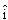+ 2xy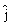– yz2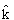at a point (1,1,1 ) is equal to
(a) 7
(b) 4
(c) 3
(d) 0
Ans: (c)

Question: If three coins are tossed simultaneously, the probability of getting at least one head
(a) 1/8
(b) 3/8
(c) 1/2
(d) 7/8
Ans: (d)

Question: If a closed system is undergoing an irreversible process, the entropy of the system
(a) Must increase
(b) Always remains constant
(c) Must decrease
(d) Can increase, decrease or remain constant
Ans: (d)

Question: A frictionless piston-cylinder device contains a gas initially at 0.8MPa and 0.015 m. It expands quasi-statically at constant temperature to a final volume of 0.030 m. The work output (in kJ) during this process will be
(a) 8.32
(b) 12.00
(c) 554.67
(d) 8320.00
Ans: (a)

Question: In an ideal vapour compression refrigeration cycle, the specific enthalpy of refrigerant (in kJ/kg) at the following states is given as:
Inlet of condenser: 283
Exit of condenser: 116
Exit of evaporator: 232
The COP of this cycle is
(a) 2.27
(b) 2.75
(c) 3.27
(d) 3.75
Ans: (a)

Question: If the principal stresses in a plane stress problem, are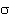1 = 100MPa,2 = 40MPa , the magnitude of the maximum shear stress (in MPa) will be
(a) 60
(b) 50
(c) 30
(d) 20
Ans: (c)

Question: A simple quick return mechanism is shown in the figure. The forward to return ratio of the quick return mechanism is 2 : 1. If the radius of the crank O1P is 125 mm, then the distance ‘d’ (in mm) between the crank centre to lever pivot centre point should be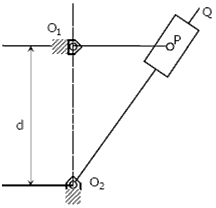(a) 144.3
(b) 216.5
(c) 240.0
(d) 250.0
Ans: (d)

Question: The rotor shaft of a large electric motor supported between short bearings at both deflection of 1.8mm in the middle of the rotor. Assuming the rotor to be perfectly balanced and supported at knife edges at both the ends, the likely critical speed (in rpm) of the shaft is
(a) 350
(b) 705
(c) 2810
(d) 4430
Ans: (b)

Question: Friction at the tool-chip interface can be reduced by
(a) decreasing the rake angle
(b) increasing the depth of cut
(c) decreasing the cutting speed
(d) increasing the cutting speed
Ans: (d)

Question: Which of the following forecasting methods takes a fraction of forecast error into account for the next period forecast?
(a) simple average method
(b) moving average method
(c) weighted moving average method
(d) exponential smoothening method
Ans: (d)

Question: The Blasius equation,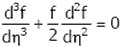is a
(a) Second order nonlinear ordinary differential equation
(b) Third order nonlinear ordinary differential equation
(c) Third order linear ordinary differential equation
(d) Mixed order nonlinear ordinary differential equation
Ans: (b)

Question: There are two points P and Q on a planar rigid body. The relative velocity between the two points
(a) should always be along PQ
(b) Can be oriented along any direction
(c) should always be perpendicular to PQ
(d) should be along QP when the body undergoes pure translation
Ans: (c)

Question: Which of the following statements is INCORRECT?
(a) Grashof’s rule states that for a planar crank-rocker four bar mechanism, the sum of the shortest and longest link lengths cannot be less than the sum of the remaining two link lengths.
(b) Inversions of a mechanism are created by fixing different links one at a time.
(c) Geneva mechanism is an intermittent motion device
(d) Gruebler’s criterion assumes mobility of a planar mechanism to be one.
Ans: (a)

Question: Tooth interference in an external involute spur gear pair can be reduced by
(a) decreasing center distance between gear pair
(b) decreasing module
(c) decreasing pressure angle
(d) increasing number of gear teeth
Ans: (d)

Question: The maximum velocity of a one-dimensional incompressible fully developed viscous flow, between two fixed parallel plates, is 6ms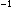. The mean velocity (in ms) of the flow is
(a) 2
(b) 3
(c) 4
(d) 5
Ans: (c)

Question: A turbo-charged four-stroke direct injection diesel engine has a displacement volume of 0.0259 m(25.9 litres). The engine has an output of 950 kW at 2200 rpm. The mean effective pressure in MPa is closest to
(a) 2
(b) 1
(c) 0.2
(d) 0.1
Ans: (a)

Question: One kilogram of water at room temperature is brought into contact with a high temperature thermal reservoir. The entropy change of the universe is
(a) equal to entropy change of the reservoir
(b) equal to entropy change of water
(c) equal to zero
(d) always positive
Ans: (d)

Question: A hydraulic turbine develops 1000kW power for a head of 40m. If the head is reduced to 20m, the power developed (in kW) is
(a) 177
(b) 354
(c) 500
(d) 707
Ans: (b)

Question: The material property which depends only on the basic crystal structure is
(a) fatigue strength
(b) work hardening
(c) fracture strength
(d) elastic constant
Ans: (c)

Question: In a gating system, the ratio 1 : 2 : 4 represents
(a) sprue base area: runner area: ingate area
(b) pouring basin area: ingate area: runner area
(c) sprue base area: ingate area: casting area
(d) runner area: ingate area: casting area
Ans: (a)

Question: In a CNC program block, N002 G02 G91 X40 Z40…, G02 AND G91 refer to
(a) circular interpolation in counterclockwise direction and incremental dimension
(b) circular interpolation in counterclockwise direction and absolute dimension
(c) circular interpolation in clockwise direction and incremental dimension
(d) circular interpolation in clockwise direction and absolute dimension
Ans: (c)

Question: The demand and forecast for February are 12000 and 10275, respectively. Using single exponential smoothening method (smoothening coefficient = 0.25), forecast for the month of March is
(a) 431
(b) 9587
(c) 10706
(d) 11000
Ans: (c)

Related: Mechanical Engineering

Question: Simplex method of solving linear programming problem uses
(a) all the points in the feasible region
(b) only the corner points of the feasible region
(c) intermediate points within the infeasible region
(d) only the interior points in the feasible region
Ans: (b)

Question: The maximum possible draft in cold rolling of sheet increases with the
(a) Increase in coefficient of friction
(b) Decrease in coefficient of friction
(d) Increase in roll velocity
Ans: (a)

Question: The operation in which oil is permeated into the pores of a powder metallurgy product is known as
(a) Mixing
(b) Sintering
(c) Impregnation
(d) Infiltration
Ans: (c)

Questions carrying Two Marks Each

Question: A torque T is applied at the free end of a stepped rod of circular cross-sections as shown in the figure. The shear modulus of the material of the rod is G. The expression for d to produce an angular twistat the free end is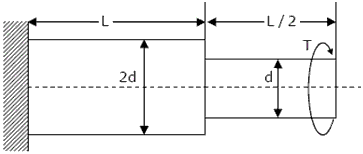(a)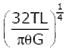(b)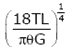(c)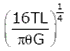(d)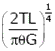Ans. (b)

Question: Figure shows the schematic for the measurement of velocity of air (density = 1.2 kg/m) through a constant–area duct using a pitot tube and a water-tube manometer. The differential head of water (density = 1000 kg/m) in the two columns of the manometer is 10mm. Take acceleration due to gravity as 2 9.8m/ s . The velocity of air in m/s is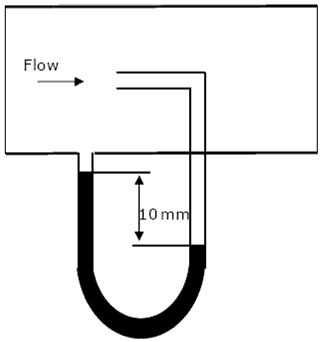(a) 6.4
(b) 9.0
(c) 12.8
(d) 25.6
Ans: (c)

Question: The values of enthalpy of steam at the inlet and outlet of a steam turbine in a Rankine cycle are 2800kJ/kg and 1800kJ/kg respectively. Neglecting pump work, the specific steam consumption in kg/kW-hour is
(a) 3.60
(b) 0.36
(c) 0.06
(d) 0.01
Ans: (a)

Question: For the four-bar linkage shown in the figure, the angular velocity of link AB is 1 rad/s. the length of link CD is 1.5 times the length of link AB. In the configuration shown, the angular velocity of link CD in rad/s is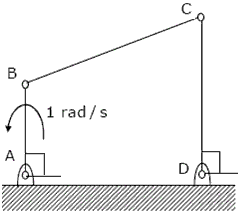(a) 3
(b) 3/2
(c) 1
(d) 2/3
Ans: (d)

Question: An unbiased coin is tossed five times. The outcome of each toss is either a head or a tail. The probability of getting at least one head is
(a) 1/32
(b) 13/32
(c) 16/32
(d) 31/32
Ans: (d)

Question: A mass of 1kg is attached to two identical springs each with stiffness k = 20kN/m as shown in the figure. Under frictionless condition, the natural frequency of the system in Hz is close to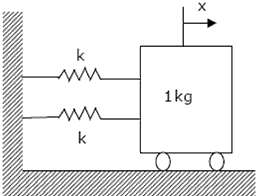(a) 32
(b) 23
(c) 16
(d) 11
Ans: (a)

Question: The shear strength of a sheet metal is 300MPa. The blanking force required to produce a blank of 100mm diameter from a 1.5 mm thick sheet is close to
(a) 45 kN
(b) 70 kN
(c) 141 kN
(d) 3500 kN
Ans: (c)

Question: The crank radius of a single–cylinder I. C. engine is 60mm and the diameter of the cylinder is 80mm. The swept volume of the cylinder in cmis
(a) 48
(b) 96
(c) 302
(d) 603
Ans: (d)

Question: An ideal Brayton cycle, operating between the pressure limits of 1 bar and 6 bar, has minimum and maximum temperatures of 300K and 1500K. The ratio of specific heats of the working fluid is 1.4. The approximate final temperatures in Kelvin at the end of the compression and expansion processes are respectively
(a) 500 and 900
(b) 900 and 500
(c) 500 and 500
(d) 900 and 900
Ans: (a)

Question: A disc of mass m is attached to a spring of stiffness k as shown in the figure. The disc rolls without slipping on a horizontal surface. The natural frequency of vibration of the system is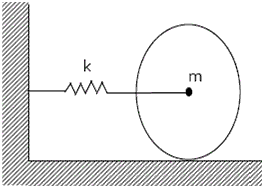(a)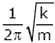(b)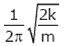(c)(d)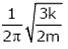Ans. (c)

Question: A 1 kg block is resting on a surface with coefficient of friction µ = 0.1. A force of 0.8N is applied to the block as shown in figure. The friction force is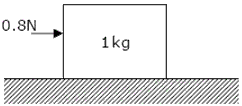(a) 0 N
(b) 0.8 N
(c) 0.98 N
(d) 1.2 N
Ans: (b)

Question: A single–point cutting tool with 12rake angle is used to machine a steel work–piece. The depth of cut, i.e. uncut thickness is 0.81 mm. The chip thickness under orthogonal machining condition is 1.8 mm. The shear angle is approximately
(a) 22(b) 26(c) 56(d) 76Ans: (b)

Question: Match the following non-traditional machining processes with the corresponding material removal mechanisms :

 Machining process Mechanism of material removal P. Chemical machining 1. Erosion Q. Electro-chemical machining 2. Corrosive reaction R. Electro – discharge machining 3. Ion displacement S. Ultrasonic machining 4. Fusion and vaporization

(a) P – 2, Q – 3, R – 4, S – 1
(b) P – 2, Q – 4, R – 3, S – 1
(c) P – 3, Q – 2, R – 4, S – 1
(d) P – 2, Q – 3, R – 1, S – 4
Ans: (a)

Question: A cubic casting of 50mm side undergoes volumetric solidification shrinkage and volumetric solid contraction of 4% and 6% respectively. No. riser is used. Assume uniform cooling in all directions. The side of the cube after solidification and contraction is
(a) 48.32 mm
(b) 49.90 mm
(c) 49.94 mm
(d) 49.96 mm
Ans: (a)

Question: In a parallel flow heat exchanger operating under steady state, the heat capacity rates (product of specific heat at constant pressure and mass flow rate) of the hot and cold fluid are equal. The hot fluid, flowing at 1kg/s with Cp = 4kJ/kgK, enters the heat exchanger at 102°C while the cold fluid has an inlet temperature of 15°C. The overall heat transfer coefficient for the heat exchanger is estimated to be 1kW/mK and the corresponding heat transfer surface area is 5m. Neglect heat transfer between the heat exchanger and the ambient. The heat exchanger is characterized by the following relation: 2= 1 – exp (-2NTU) . The exit temperature (in °C) for the cold fluid is
(a) 45
(b) 55
(c) 65
(d) 75
Ans: (b)

Question: In an air-standard Otto cycle, the compression ratio is 10. The condition at the beginning of the compression process is 100 kPa and 27°C. Heat added at constant volume is 1500 kJ/kg, while 700 kJ/kg of heat is rejected during the other constant volume process in the cycle. Specific gas constant for air = 0.287 kJ/kgK. The mean effective pressure (in kPa) of the cycle is
(a) 103
(b) 310
(c) 515
(d) 1032
Ans: (d)

Question: An irreversible heat engine extracts heat from a high temperature source at a rate of 100kW and rejects heat to a sink at a rate of 50kW. The entire work output of the heat engine is used to drive a reversible heat pump operating between a set of independent isothermal heat reservoirs at 17°C and 75°C. The rate (in kW) at which the heat pump delivers heat to its high temperature sink is
(a) 50
(b) 250
(c) 300
(d) 360
Ans: (c)

Question: Water at 25°C is flowing through a 1.0 km long G.I pipe of 200 mm diameter at the rate of 0.07 m/s3. If value of Darcy friction factor for this pipe is 0.02 and density of water is 1000 kg/m, the pumping power (in kW) required to maintain the flow is
(a) 1.8
(b) 17.4
(c) 20.5
(d) 41.0
Ans: (b)
Ans: (b)

Question: A solid shaft of diameter, d and length L is fixed at both the ends. A torque, T0 is applied at a distance, L/4 from the left end as shown in the figure given below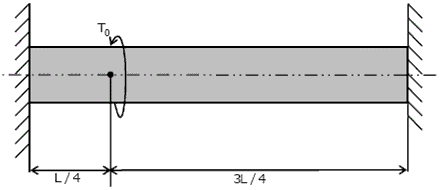The maximum shear stress in the shaft is

 (a)(b)(c)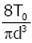(d)Ans. (b)

Question: An automotive engine weighing 240kg is supported on four springs with linear characteristics. Each of the front two springs have a stiffness of 16MN/m while the stiffness of each rear spring is 32MN/m. The engine speed (in rpm), at which resonance is likely to occur, is
(a) 6040
(b) 3020
(c) 1424
(d) 955
Ans: (b)

Question: A uniform rigid rod of mass M and length L is hinged at one end as shown in the adjacent figure. A force P is applied at a distance of 2L/3 from the hinge so that the rod swings to the right. The reaction at the hinge is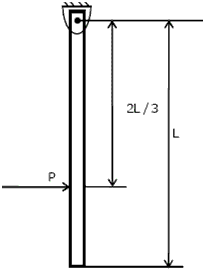(a) -P
(b) 0
(c) P/3
(d) 2P/3
Ans: (b)

Question: Match the approaches given below to perform stated kinematics / dynamics analysis of machine.

 Analysis Approach P. Continuous relative rotation 1. D’ Alembert principle Q. velocity and acceleration 2. Grubler’s criterion R. Mobility 3. Grashoff’s law S. dynamic-static analysis 4. Kennedy’s theorem

(a) P-1,Q-2,R-3,S-4
(b) P-3,Q-4,R-2,S-1
(c) P-2,Q-3,R-4,S-1
(d) P-4,Q-2,R-1,S-3
Ans: (b)

Common Data Questions: 48 & 49

In an experimental set-up, air flows between two stations P and Q adiabatically. The direction of flow depends on the pressure and temperature conditions maintained at P and Q. The conditions at station P are 150kPa and 350K. The temperature at station Q is 300K.

The following are the properties and relations pertaining to air:
Specific heat at constant pressure, Cp = 1.005kJ /kgK;
Specific heat at constant volume, Cv = 0.718kJ /kgK;
Characteristic gas constant, R = 0.287kJ /kgK
Enthalpy,  ho = c T
Internal energy, uv = c T

Question: If the air has to flow from station P to station Q, the maximum possible value of pressure in kPa at station Q is close to
(a) 50
(b) 87
(c) 128
(d) 150
Ans: (b)

Question: If the pressure at station Q is 50 kPa, the change in entropy (SQ -SP) in kJ/kgK is
(a) -0.155
(b) 0
(c) 0.160
(d) 0.355
Ans: (c)

Common Data Questions

Radiative heat transfer is intended between the inner surfaces of two very large isothermal parallel metal plates. While the upper plate (designated as plate 1) is a black surface and is the warmer one being maintained at 727ºC, the lower plate (plate 2) is a diffuse and gray surface with an emissivity of 0.7 and is kept at 227ºC. Assume that the surfaces are sufficiently large to form a two-surface enclosure and steady state conditions to exist. Stefan Boltzmann constant is given as 5.67×10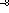W/mKQuestion: The irradiation (in kW/m) for the upper plate (plate 1) is
(a) 2.5
(b) 3.6
(c) 17.0
(d) 19.5
Ans: (a)

Question: If plate 1 is also a diffuse and gray surface with an emissivity value of 0.8, the net radiation heat exchange (in kW/m) between plate 1 and plate 2 is
(a) 17.0
(b) 19.0
(c) 23.0
(d) 31.7
Ans: (d)

In a machining experiment, tool life was found to vary with the cutting speed in the following manner:

 Cutting speed (m/min) Tool life (minutes) 60 81 90 36

Question: The exponent (n) and constant (k) of the Taylor’s tool life equation are
(a) n = 0.5 and k = 540
(b) n = 1 and k = 4860
(c) n = -1 and k = 0.74
(d) n = −0.5 and k = 1.155
Ans: (a)

Question: What is the percentage increase in tool life when the cutting speed is halved?
(a) 50%
(b) 200%
(c) 300%
(d) 400%
Ans: (c)

The temperature and pressure of air in a large reservoir are 400K and 3 bar respectively. A converging–diverging nozzle of exit area 0.005mis fitted to the wall of the reservoir as shown in the figure. The static pressure of air at the exit section for isentropic flow through the nozzle is 50kPa. The characteristic gas constant and the ratio of specific heats of air are 0.287kJ/kgK and 1.4 respectively.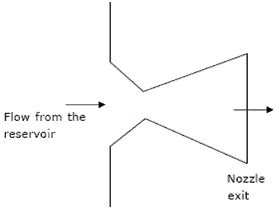Question: The density of air in kg / mat the nozzle exit is
(a) 0.560
(b) 0.600
(c) 0.727
(d) 0.800
Ans: (c)

Question: The mass flow rate of air through the nozzle in kg/s is
(a) 1.30
(b) 1.77
(c) 1.85
(d) 2.06
Ans: (d)

Questions carrying One Mark Each

Question: Which of the following options is the closest in the meaning to the word below:
Inexplicable
(a) Incomprehensible
(b) Indelible
(c) Inextricable
(d) Infallible
Ans: (a)

Question: Choose the word from the options given below that is most nearly opposite in meaning to the given word:
Amalgamate
(a) Merge
(b) Split
(c) Collect
(d) Separate
Ans: (b)

Question: Choose the most appropriate word from the options given below to complete the following sentence.

If you are trying to make a strong impression on your audience, you cannot do so by being understated, tentative or ______.
(a) Hyperbolic
(b) Restrained
(c) Argumentative
(d) Indifferent
Ans: (b)

Question: If in a certain language, 943 is coded as BED and 12448 is coded as SWEET, how is 492311 coded in that language ?
(a) EDSWBS
(b) TSWBDD
(c) DSWTEE
(d) EBWDSS
Ans: (d)

Question: Choose the most appropriate word(s) from the options given below to complete the following sentence.

I contemplated ____ Singapore for my vacation but decided against it.
(a) To visit
(b) having to visit
(c) visiting
(d) for a visit
Ans: (c)

Questions carrying Two Marks Each

Question: Few school curricula include a unit on how to deal with bereavement and grief, and yet all students at some point in their lives suffer from losses through death and parting.

Based on the above passage which topic would not be included in a unit on bereavement?
(a) how to write a letter of condolence
(b) what emotional stages are passed through in the healing process
(c) what the leading causes of death are
(d) how to give support to a grieving friend
Ans: (c)

Question: The variable cost (V) of manufacturing a product varies according to the equation V= 4q, where q is the quantity produced. The fixed cost (F) of production of same product reduces with q according to the equation F = 100/q. How many units should be produced to minimize the total cost (V+F)?
(a) 5
(b) 4
(c) 7
(d) 6
Ans: (a)

Question: A container originally contains 10 litres of pure spirit. From this container 1 litre of spirit is replaced with 1 litre of water. Subsequently, 1 litre of the mixture is again replaced with 1 litre of water and this process is repeated one more time.

How much spirit is now left in the container?
(a) 7.58 litres
(b) 7.84 litres
(c) 7 litres
(d) 7.29 litres
Ans: (d)

Question: A transporter receives the same number of orders each day. Currently, he has some pending orders (backlog) to be shipped. If he uses 7 trucks, then at the end of the 4th day he can clear all the orders. Alternatively, if he uses only 3 trucks, then all the orders are cleared at the end of the 10th day. What is the minimum number of trucks required so that there will be no pending order at the end of the 5th day?
(a) 4
(b) 5
(c) 6
(d) 7
Ans: (c)

Question: P, Q, R and S are four types of dangerous microbes recently found in a human habitat. The area of each circle with its diameter printed in brackets represents the growth of a single microbe surviving human immunity system within 24 hours of entering the body. The danger to human beings varies proportionately with the toxicity, potency and growth attributed to a microbe shown in the figure below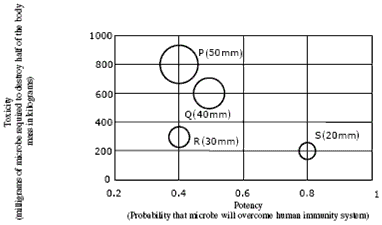A pharmaceutical company is contemplating the development of a vaccine against the most dangerous microbe. Which microbe should the company target in its first attempt?
(a) P
(b) Q
(c) R
(d) S
Ans: (d)